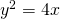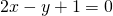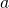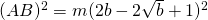# Random Questions from 2016 Prelims #4VJC P1 Q6A curve has equationand a linehas equationas shown above.is a fixed point on C and A is an arbitrary point on. State the geometrical relationship between the line segment AB andis the distance from B to A is the least.

Taking the coordinates of A as, find an equation relatingandfor which AB is the least.

Deduce that when AB is the least,whereis a constant to be found. Hence or otherwise, find the coordinates of the point on C that is nearest on.

Not readable? Change text.